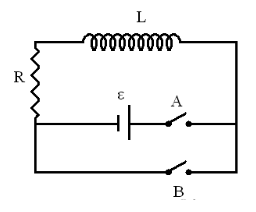# Problem: An RL circuit is shown on the right. L = 2.2 J, R = 258 Ω, ε = 4.5 V.  Switch A is closed at t = 0.(a) Calculate the numerical value of I at t = 0.1 s in amperes.(b) Calculate the numerical value of I at t = L/R s in amperes.(c) Calculate the numerical value of I, in amperes, when t goes to infinity.

###### FREE Expert Solution

Current:

Part (a)

i(0.1s) = (ε/R)(1 - e(-R/L)t) = (4.5/258)(1 - e(-258/2.2)0.1) = 0.0174 A

83% (289 ratings)###### Problem Details

An RL circuit is shown on the right. L = 2.2 J, R = 258 Ω, ε = 4.5 V.  Switch A is closed at t = 0.
(a) Calculate the numerical value of I at t = 0.1 s in amperes.
(b) Calculate the numerical value of I at t = L/R s in amperes.
(c) Calculate the numerical value of I, in amperes, when t goes to infinity.